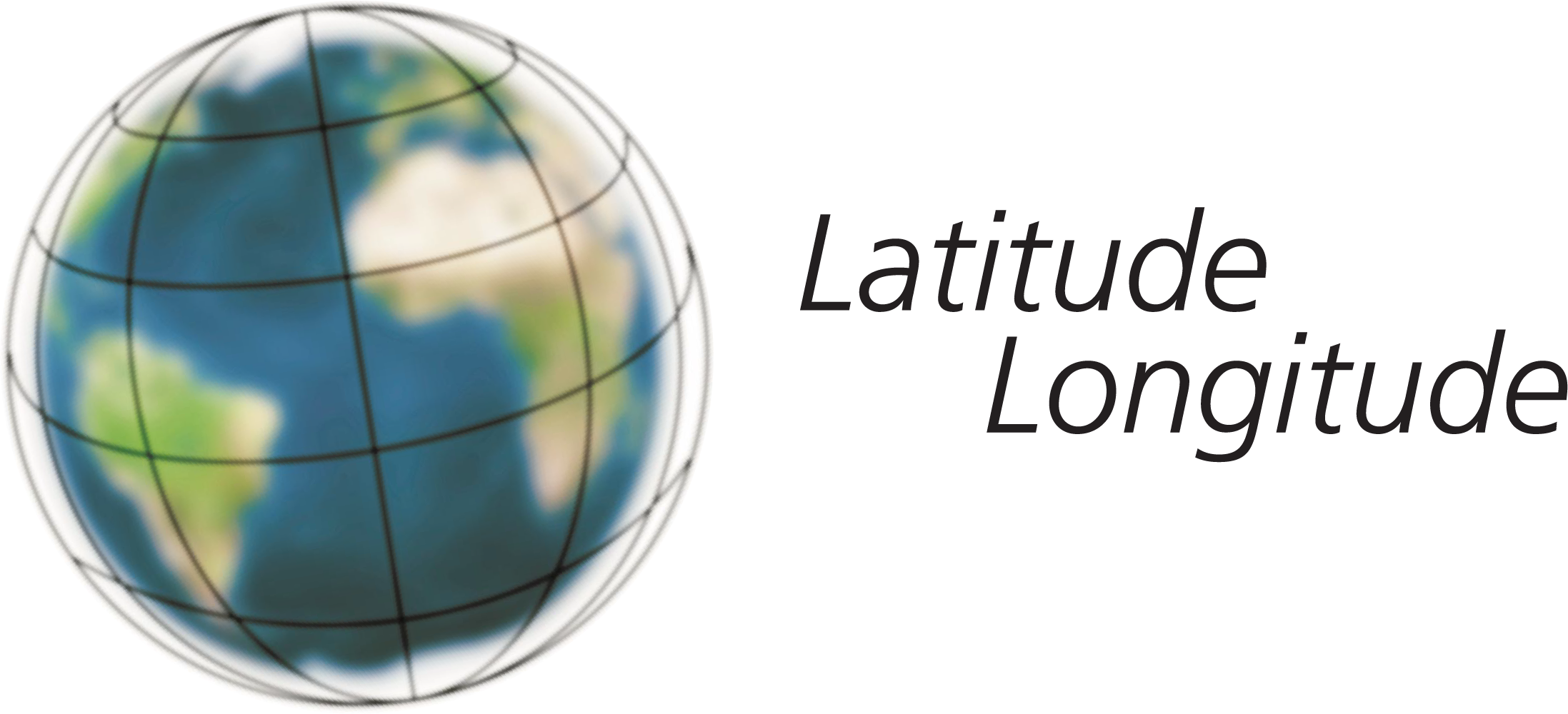## MEASURING ANGLES AND WIDTHS

Notebook "Where are you?"An angle is the measurement of one full line of a circumference. For example, if you trace a full line along the surface of an orange or a ball with your finger, when you arrive at the point you started from you have gone a 360º angle. In other words, you circled an angle of 360º on the ball or the orange.

### Experiment

Cut out the protractor. A protractor is a special ruler which is used to measure angles on a circumference.

You can now use your protractor to trace a semi-circumference and measure the angles when you follow the circumference from the right-hand side. The black dot on the protractor is the centre of the circumference.The diagram shows angles of 0 degrees, 30 degrees, 60 degrees and 90 degrees. Semi-circumference corresponds to a 180º angle and a full circumference corresponds to 360 degrees. When the Sun is at its highest in the sky its angle above the horizon line is larger. At sunrise and sunset, it is closer to the horizon and its angle is much smaller. The 0 degree angle is the horizon line.Get your protractor and a pencil.

Line up your protractor with the Sun so that the shadow of the protractor is as thin as possible. Put the point of the pencil on top of the protractor in such a way that its shadow falls through the black dot on the protractor. The point of the pencil shows a number on the scale. This is the angle of the Sun above the horizon.